### Computational Method

#### Canonical Analysis

For each response variable, the model can be written in the form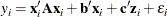whereis the ith observation of the response variable.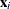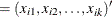are the k factor variables for the ith observation.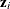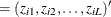are the L covariates, including the intercept term.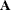is the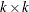symmetrized matrix of quadratic parameters, with diagonal elements equal to the coefficients of the pure quadratic terms in the model and off-diagonal elements equal to half the coefficient of the corresponding crossproduct.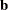is the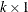vector of linear parameters.is the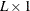vector of covariate parameters, one of which is the intercept.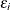is the error associated with the ith observation. Tests performed by PROC RSREG assume that errors are independently and normally distributed with mean zero and variance.

The parameters in,, andare estimated by least squares. To optimizewith respect to, take partial derivatives, set them to zero, and solve: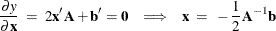You can determine if the solution is a maximum or minimum by looking at the eigenvalues of:

If the eigenvalues…

then the solution is…

are all negative

a maximum

are all positive

a minimum

have mixed signs

contain zeros

in a flat area

#### Ridge Analysis

If the largest eigenvalue is positive, its eigenvector gives the direction of steepest ascent from the stationary point; if the largest eigenvalue is negative, its eigenvector gives the direction of steepest descent. The eigenvectors corresponding to small or zero eigenvalues point in directions of relative flatness.

The point on the optimum response ridge at a given radius R from the ridge origin is found by optimizing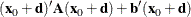over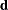satisfying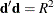, where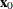is thevector containing the ridge origin andandare as previously discussed. By the method of Lagrange multipliers, the optimalhas the form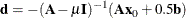whereis theidentity matrix andis chosen so that. There can be several values ofthat satisfy this constraint; the correct one depends on which sort of response ridge is of interest. If you are searching for the ridge of maximum response, then the appropriateis the unique one that satisfies the constraint and is greater than all the eigenvalues of. Similarly, the appropriatefor the ridge of minimum response satisfies the constraint and is less than all the eigenvalues of. (See Myers and Montgomery (1995) for details.)